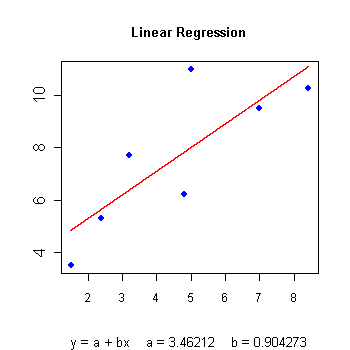•http://facebook.com/
•https://www.google.com/accounts/o8/id
•https://me.yahoo.comCOST (GBP)3.500.000

# Linear

viewed 16616 times and licensed 1118 times
Calculates the linear regression parameters and evaluates the regression line at arbitrary abscissas
Controller: CodeCogsC++

## Class Linear

Linear regression is a method to best fit a linear equation (straight line) of the form to a collection of points , where is the slope and the intercept on the axis.

The algorithm basically requires minimisation of the sum of the squared distance from the data points to the proposed line. This is achieved by calculating the derivative with respect to a and b and setting these to zero.

Let us define the following

Then the slope is the intercept on the Y axis

Below you will find the regression graph for a set of arbitrary points, which were also used in the forthcoming example. The regression line, displayed in red, has been calculated using this class.### Example 1

The following example displays the slope, Y intercept and regression coefficient for a certain set of 7 points.
#include <codecogs/maths/approximation/regression/linear.h>
#include <iostream>
#include <iomanip>
using namespace std;

int main()
{
double x = { 1.5, 2.4, 3.2, 4.8,  5.0, 7.0,  8.43 };
double y = { 3.5, 5.3, 7.7, 6.2, 11.0, 9.5, 10.27 };

Maths::Regression::Linear A(7, x, y);

cout << "    Slope = " << A.getSlope() << endl;
cout << "Intercept = " << A.getIntercept() << endl << endl;

cout << "Regression coefficient = " << A.getCoefficient() << endl;

cout << endl << "Regression line values" << endl << endl;
for (double i = 0.0; i <= 3; i += 0.6)
{
cout << "x = " << setw(3) << i << "  y = " << A.getValue(i);
cout << endl;
}
return 0;

}
Output:
Slope = 0.904273
Intercept = 3.46212

Regression coefficient = 0.808257

Regression line values

x =   0  y = 3.46212
x = 0.6  y = 4.00469
x = 1.2  y = 4.54725
x = 1.8  y = 5.08981
x = 2.4  y = 5.63238
x =   3  y = 6.17494

### Authors

Lucian Bentea (August 2005)
##### Source Code

Source code is available when you agree to a GP Licence or buy a Commercial Licence.

Not a member, then Register with CodeCogs. Already a Member, then Login.

## Members of Linear

#### Linear

 Linear( int n double* x double* y )[constructor]
Initializes the class by calculating the slope, intercept and regression coefficient based on the given constructor arguments.

### Note

The slope should not be infinite.
 n The number of initial points in the arrays x and y x The x-coordinates of points y The y-coordinates of points

#### GetValue

 doublegetValue( double x )
 x the abscissa used to evaluate the linear regression function

#### GetCoefficient

 doublegetCoefficient( )
The regression coefficient indicated how well linear regression fits to the original data. It is an expression of error in the fitting and is defined as:

This varies from 0 (no linear trend) to 1 (perfect linear fit). If and , then r is considered to be equal to 1.

## Linear Once

 doubleLinear_once( int n double* x double* y double a )
This function implements the Linear class for one off calculations, thereby avoid the need to instantiate the Linear class yourself.

### Example 2

The following graph fits a straight line to the following values:
x = 1  y = 0.22
x = 2  y = 0.04
x = 3  y = -0.13
x = 4  y = -0.17
x = 5  y = -0.04
x = 6  y = 0.09
x = 7  y = 0.11
There is an error with your graph parameters for Linear_once with options n=7 x="1 2 3 4 5 6 7" y="0.22 0.04 -0.13 -0.17 -0.04 0.09 0.11" a=1:7 .input

Error Message:Function Linear_once failed. Ensure that: Invalid C++

### Parameters

 n The number of initial points in the arrays x and y x The x-coordinates of points y The y-coordinates of points a The x-coordinate for the output location

### Returns

the interpolated y-coordinate that corresponds to a.
##### Source Code

Source code is available when you agree to a GP Licence or buy a Commercial Licence.

Not a member, then Register with CodeCogs. Already a Member, then Login.

Last Modified: 21 Mar 13 @ 23:17     Page Rendered: 2022-03-14 17:44:28